## BayaderaBayes + Clojure + GPU

### Dragan Djuric

[email protected]

## A little background

• Clojure
• GPU computing
• Bayesian Data Analysis

### Clojure - a modern lisp

• Dynamic and fast
• First-class functions
• Great abstractions and data structures
• Many useful libraries
• Even more experimental libraries
• ClojureScript in browsers, node etc.

### Are Java and Clojure good at number crunching?

• Good? maybe.
• Great? NO!
• We can make it great!

### CPU is not so great either!

• R, Python? Even worse than Java.
• C? complicated, verbose platform-specific optimizations.
• CPU? too beefed-up. Burns as the Sun!

### GPU has a lot to offer …at a price

• many dumb computing units
• but, power-efficient for number crunching
• hardware support for massive parallelism
• faster and cheaper each year
• notoriously difficult to program

### Uncomplicate

Fluokitten
ClojureCL
take control of the GPU and CPU from Clojure
Neanderthal
optimized vectors and matrices on CPU and GPU
high performance Bayesian statistics and data analysis on the GPU

### What does all this has to do with Bayes?

• BDA is conceptually simple
• Real models must be calculated numerically
• MCMC sampling and related methods
• In higher dimensions, requires huge samples
• Analyses typically run in minutes, hours, weeks

.

• Good: Clojure and GPU FTW
• Challenge: MCMC is sequential

## A Bayesian Data Analysis Primer

How to know something we cannot observe?

• Probabilities of all answers (prior)
• Probability of measured data (evidence and likelihood)
• Calculate "backwards", using Bayes' rule and get
\begin{equation*} \Pr(H|D) = \frac{\Pr(D|H)\times \Pr(H)}{\Pr(D)} \end{equation*}

### Doing Bayesian Data Analysis

• John Kruschke
• Excellent tutorial, even for beginners
• Real-world examples through the book!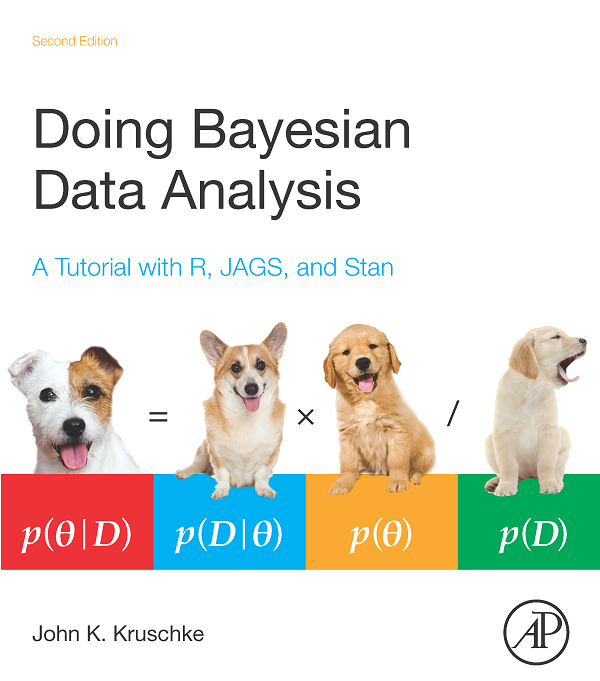### Hello World: Fair or Trick coin?

• Coin bias, tendency to fall on head: θ
• We can not measure θ directly
• We can only know sample data (D)
• θ is general; θ1, θ2, …, θn are specific values
• D is general; 3 heads out of 10 specific

By Bayes' rule:

\begin{equation*} \Pr(\theta|D) = \frac{\Pr(D|\theta)\times \Pr(\theta)}{\Pr(D)} \end{equation*}

### Analytical solution

Likelihood:

Pr(z,N|θ) = θz (1 - θ)N-z

(conjugate) prior

Pr(θ|a,b) = beta(θ|a,b) = θ(a-1)(1-θ)(b-1)/B(a,b)

Posterior, conveniently:

Pr(θ|z,N) = beta(θ|z+a, N - z + b)

### Example from Kruschke's book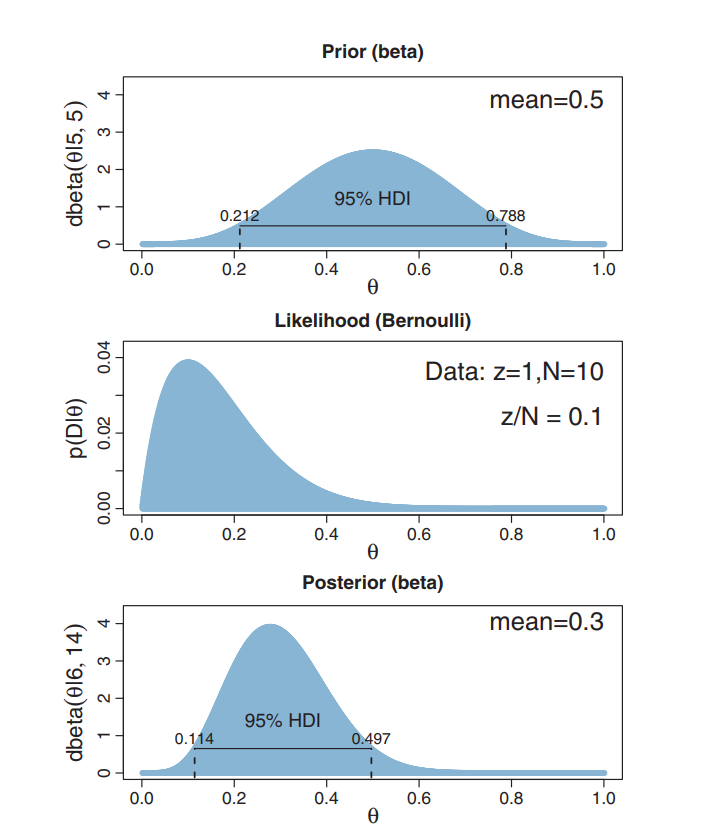### Easy computation

Q: What is Pr(0.4<θ<0.6)?

A: Pr(θ<0.6) - Pr(θ<0.4)

(let [a-prior 5
b-prior 5
z 1
N 10
a-post (+ a-prior z)
b-post (+ b-prior (- N z))]

(- (beta-cdf a-post b-post 0.6)
(beta-cdf a-post b-post 0.4)))


### Never happens in practice

• Only the simplest models are "nice"
• The curse of dimensionality

• a Clojure library
• highly opinionated - Bayesian
• probabilistic
• need to NOT be super slow - thus GPU
• actually is the fastest I have seen
• use cases:
• Bayesian data analysis (more stats-oriented)
• a foundation for machine learning algorithms
• lots of statistical number crunching
• risk assessment, decision analysis, etc.

• programmers are the first-class citizens
• not a "me too, just in Clojure"
• different and better (for what I want to do)
• for interactive and server use

### HARD to compute

Usually:

\begin{equation*} \Pr(\vec{h}|\vec{d}) = \frac{\prod_i f(\vec{d_i},\vec{h})\times g(\vec{h})}{\idotsint \prod_i f(\vec{d_i},\vec{h})\,d \vec{h}} \end{equation*}

computationally:

\begin{equation*} answer = \frac{hard\times acceptable}{impossible} \end{equation*}

### Markov Chain Monte Carlo (MCMC)

• a family of simulation algorithms
• draws samples from unknown probability distributions
• (enough) samples approximate the distribution
\begin{equation*} \Pr(\vec{h}|\vec{d}) \propto \exp \left(\sum_i \log f(\vec{d_i},\vec{h}) + \log g(\vec{h})\right) \end{equation*}

computationally:

\begin{equation*} answer \propto zillions \times (hard\times acceptable) \end{equation*}

## The coin example through simulation

• MCMC not necessary in this case
• Good as a hello world example
• Comparison with analytical solution

### Run the analysis on the GPU

(def result (atom
(let [a 5 b 5
z 1 N 10]
(with-release
[prior-dist (beta a b)
prior-sampler (sampler prior-dist)
prior-sample (dataset (sample! prior-sampler))
prior-pdf (pdf prior-dist prior-sample)
post (posterior (posterior-model (:binomial likelihoods)
(:beta distributions)))
post-dist (post (sv (op (binomial-lik-params N z) (beta-params a b))))
post-sampler (time (doto (sampler post-dist) (mix!)))
post-sample (dataset (sample! post-sampler))
post-pdf (scal! (/ 1.0 (evidence post-dist prior-sample))
(pdf post-dist post-sample))]

{:prior {:sample (native (row (p/data prior-sample) 0))
:pdf (native prior-pdf)}
:posterior {:sample (native (row (p/data post-sample) 0))
:pdf (native post-pdf)}})))))


### Prior and posterior - samples!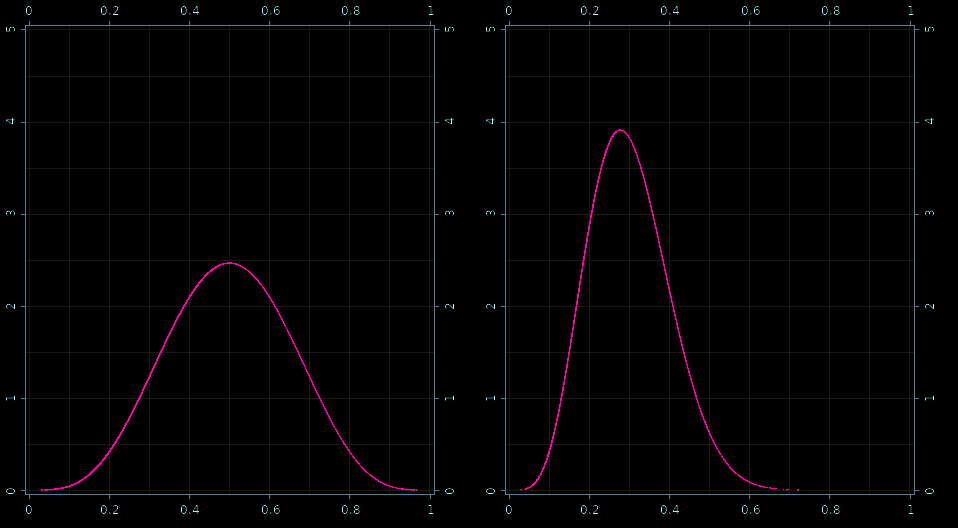## Real-World: Robust Linear Regression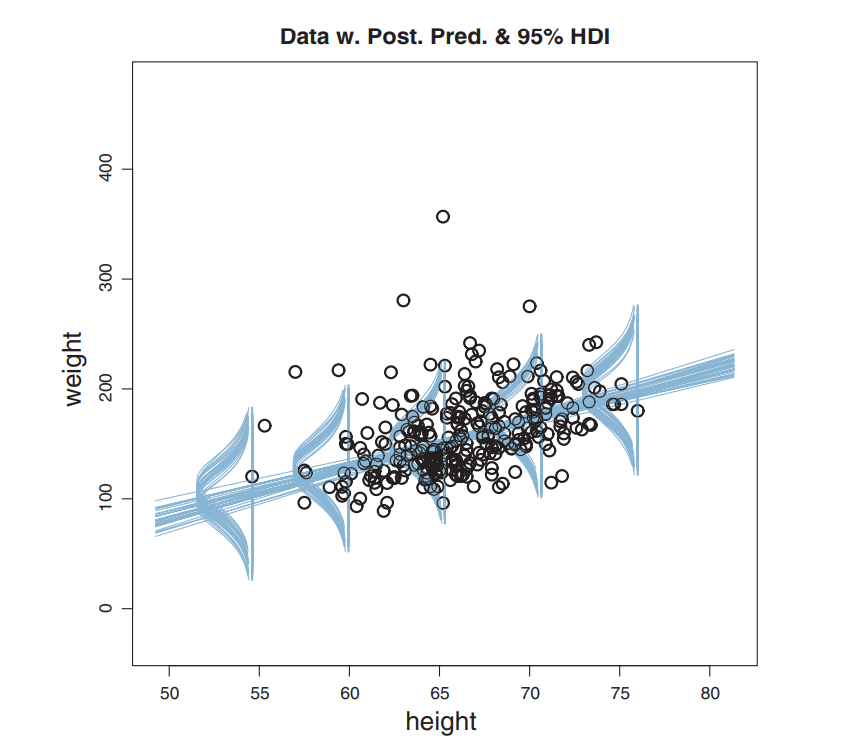### Hierarchical Model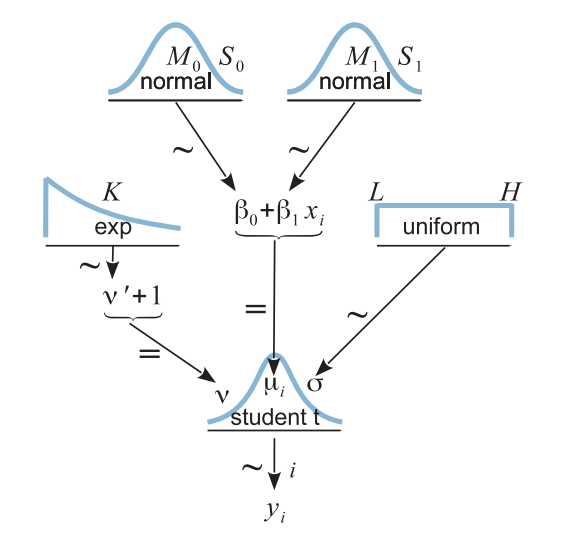### Resulting Posterior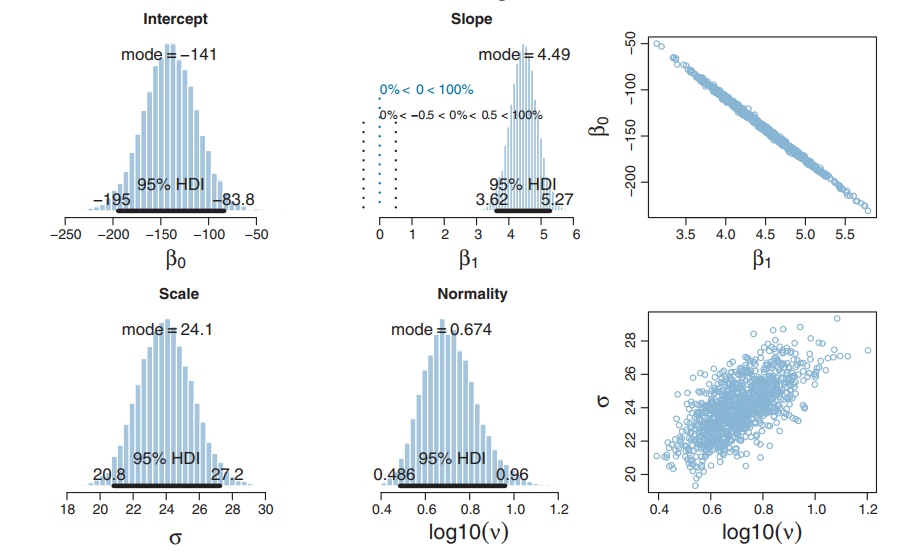(defn read-data [in-file]
(loop [c 0 data (drop 1 (csv/read-csv in-file)) hw (transient [])]
(if data
(let [[_ h w] (first data)]
(recur (inc c) (next data)
(-> hw (conj! (double (read-string h)))
(op [c] (persistent! hw)))))

(def params-300 (sv (read-data (slurp (io/file "ht-wt-data-300.csv")))))

#'user/read-data#'user/params-300


### Create a custom model

(def rlr-source (slurp (io/file "robust-linear-regression.h")))

(def rlr-prior
(cl-distribution-model [(:gaussian source-library)
(:uniform source-library)
(:exponential source-library)
(:t source-library)
rlr-source]
:name "rlr" :mcmc-logpdf "rlr_mcmc_logpdf"
:params-size 7 :dimension 4))

(defn rlr-likelihood [n]
(cl-likelihood-model rlr-source :name "rlr" :params-size n))

#'user/rlr-source#'user/rlr-prior#'user/rlr-likelihood


### Model's likelihood function

REAL rlr_loglik(__constant const REAL* params, REAL* x) {
const REAL nu = x;
const REAL b0 = x;
const REAL b1 = x;
const REAL sigma = x;
const uint n = (uint)params;
const bool valid = (0.0f < nu) && (0.0f < sigma);
if (valid) {
const REAL scale = t_log_scale(nu, sigma);
REAL res = 0.0;
for (uint i = 0; i < n; i = i+2) {
res += t_log_unscaled(nu,
b0 + b1 * params[i+1],
sigma, params[i+2])
+ scale;
}
return res;
}
return NAN;
}


### Model's prior function

REAL rlr_logpdf(__constant const REAL* params, REAL* x) {
return exponential_log(params, x - 1)
+ gaussian_log(params, params, x)
+ gaussian_log(params, params, x)
+ uniform_log(params, params, x);

}


### Running the inference on the GPU

(def result (atom
(with-release
[prior (distribution rlr-prior)
prior-dist (prior (sv 10 -100 100 5 10 0.001 1000))

post (posterior "rlr_300" (rlr-likelihood (dim params-300)) prior-dist)
post-dist (post params-300)
post-sampler (sampler post-dist {:limits (sge 2 4 [1 10 -400 100 0 20 0.01 100])})]

(mix! post-sampler {:step 384})

(histogram! post-sampler 1000)))))


### Generate posterior diagrams

(defn var-plot [] (plot2d (qa/current-applet) {:width 480 :height 240}))

(defn setup []
(q/background 0)
(q/image (show (render-histogram (var-plot) @result 1)) 0 0)
(q/image (show (render-histogram (var-plot) @result 2)) 480 0)
(q/image (show (render-histogram (var-plot) @result 3)) 0 260)
(q/image (show (render-histogram (var-plot) @result 0)) 480 260)

(q/save "robust-linear-regression.png"))

#_(q/defsketch diagrams :renderer :p2d :size :fullscreen
:setup setup :middleware [pause-on-error])


### Fine grained histograms!

Posteriors: β0, β1,σ, and ν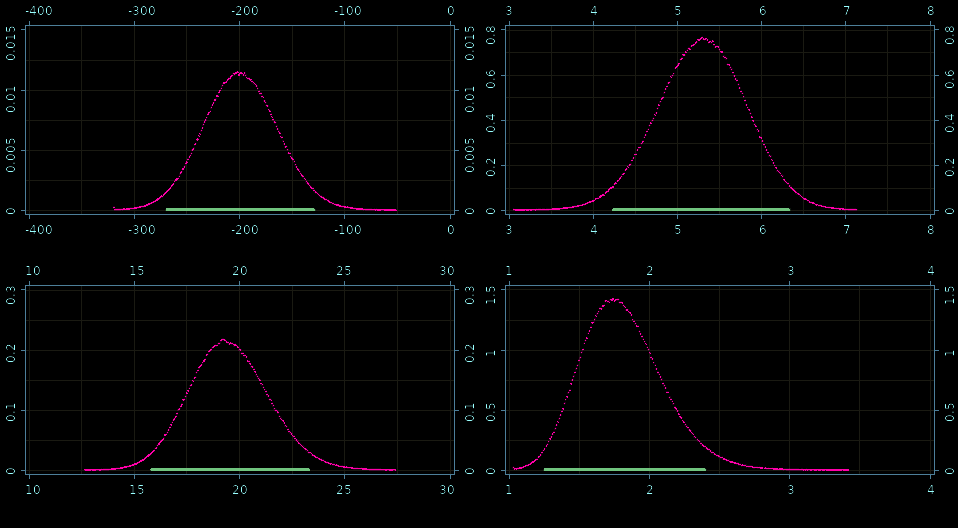## How fast is it?

• 61,208,576 samples in 267 ms.
• 4.36 ns per (computationally heavy) sample
• very precise histogram
• JAGS/Stan (state-of-the-art bayesian C++ tools)
• 20,000 samples in 180/485 seconds
• 9 ms per sample
• rough histogram
• 2,000,000 × faster per sample
• more precise results, 1000 × faster

### In real life

• 1 second vs a couple of hours
• 1 minute vs several days!
• 1 hour vs couple months/ a year

## Thank You

The presentation can be reached through my blog:

Find more at: